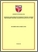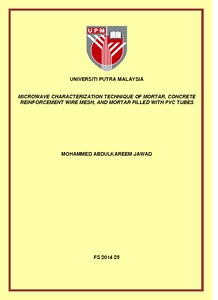# Microwave characterization technique of mortar, concrete reinforcement wire mesh, and mortar filled with pvc tubes

## Citation

Jawad, Mohammed Abdulkareem (2014) Microwave characterization technique of mortar, concrete reinforcement wire mesh, and mortar filled with pvc tubes. Masters thesis, Universiti Putra Malaysia.

## Abstract

This thesis describes a simple non-destructive free space technique to characterize Mortar, concrete reinforcement wire mesh (CRWM) and mortar filled with PVC tubes from 12 to 18 GHZ (Ku-band). This technique is simple, non-contact and precise method based on transmission and reflection properties of the samples. The measurement setup consists of a pair of transmitting and receiving lens horn antennas connected respectively to port 1 and port 2 of the Agilent N5230A PNA-L Vector Network Analyzer (VNA). The dielectric properties of the mentioned samples were measured using open ended coaxial probe. The minimum thickness of mortar structure to have a static value of dielectric constant and loss factor was found to be as 4.4 cm instead of 1.89 cm based on the manufacturers equation. The samples were placed in between the two horn antennas for the reflection and transmission coefficient measurements. The highest values of S11 and lowest S21 were found to be true for pure mortar structure whilst the opposites were found to be true for mortar filled with PVC tube. The mean S11 for a mortar, CRWM and mortar filled with tubes were 0.31, 0.27 and 0.25 whilst the mean S21 were 0.00008, 0.005 and 0.05, respectively. The curing time conditions were investigated for mortar structure at various thicknesses (1.1, 2.2, 3.3 and 4.4 cm). The transmission coefficients of all frequencies for a 1.1 cm thick sample are almost similar except for 17.5 GHZ. Reduction in S21 values at 17.5 GHZ were due to high absorption of water which coincides with its relaxation frequency. The measured results were compared with the Finite Element Method (FEM). The calculation was implemented using HFSS (High Frequency Structure Simulator). The measured dielectric constant and loss factor values were considered to be input values for the simulation to calculate (S11 and S21). Generally, the errors were found to be 0.99 for the calculated reflection coefficient (S11) and 0.013 for the calculated transmission coefficient (S21).Preview
PDF
FS 2014 25RR.pdfView Item